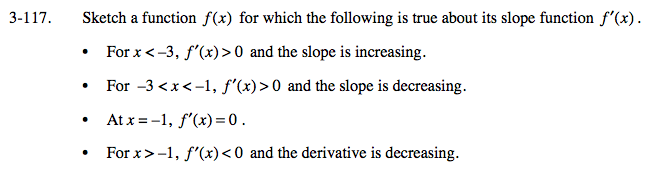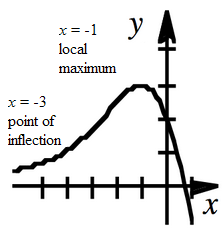### Home > CALC > Chapter 3 > Lesson 3.3.3 > Problem3-117

3-117.
1. Sketch a function f(x) for which the following is true about its slope function f ′(x). Homework Help ✎

• For x < −3, f ′(x) > 0 and the slope is increasing.

• For −3 < x < −1, f ′(x) > 0 and the slope is decreasing.

• At x = −1, f ′(x)= 0.

• For x > −1, f ′(x) < 0 and the derivative is decreasing.Be careful! The clues give information about f '(x), but you are asked to sketch f(x).

Translate: When x is greater than −3, f(x) is increasing and concave up.

Translate: When x is in between −3 and −1, f(x) is increasing and concave down.

Translate: When x is exactly −1, f(x) has reached a local maximum or minimum.Translate: When x is greater than −1, f(x) is decreasing and concave down.

Remember that this graph is based on a slope statement. Without information about the exact y-values of f(x),the entire sketch can be shifted vertically up or vertically down.